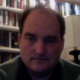## Measure Theory in Two DefinitionsMeasure theory generalizes the notion of length, area and volume to a very general topological setting. It also forms the basis of probability theory.

### Definition 1

A$\sigma$-algebra is a set$\mathcal S$ of sets which

• is closed under difference,$A - B \in {\mathcal S}$ if$A, B \in \mathcal S$,
• is closed under countable unions,$\bigcup_{n = 0}^{\infty} A_n \in \mathcal S$ if$A_n \in \mathcal S$, and
• has a unit$\mbox{I} \in \mathcal S$ such that$A \cap \mbox{I} = A$ for all$A \in \mathcal S$.

### Definition 2

A$\sigma$-additive measure is a function$\mu$ over a$\sigma$-algebra$\mathcal S$ taking non-negative values such that$\mu(\bigcup_{n=0}^{\infty} A_n) = \sum_{n=0}^{\infty} \mu(A_n)$ for pairwise disjoint sets$A_n \in \mathcal S$.

### Immediate Consequences

The closure of a$\sigma$-algebra under countable intersections follows from de Morgan’s law,$\bigcap_{n=0}^{\infty} A_n = \mbox{I} - \bigcup_{n = 0}^{\infty} (\mbox{I} - A_n)$.

The empty set$\emptyset = \mbox{I} - \mbox{I} \in \mathcal S$ is the unique zero element such that$\emptyset \cap A = \emptyset$ for all$A \in \mathcal S$. Further,$\emptyset \cup A = A$ and$\mbox{I} \cup A = \mbox{I}$ for all$A \in \mathcal S$.

A$\sigma$-algebra forms a ring, with$\cap$ as addition,$\cup$ as multiplication,$\emptyset$ as the multiplicative identity,$\mbox{I}$ as the additive identity, and$-A = \mbox{I} - A$ as additive difference.

### Constructing Lebesgue Measures

It’s not entirely trivial to show that interesting measures exist, even working over the real numbers. The easiest useful measure to construct is the Lebesgue measure over the unit interval$(0,1)$.

Start by defining the measure of a subinterval to be its length. Extend the definition to countable unions of subintervals by summation, following the definition of measure.

It remains to tidy up the some boundary cases producing zero-measure results, which isn’t entirely trivial (analysis is built on counterexamples and edge cases), but the basic idea pretty much works as is.

### 9 Responses to “Measure Theory in Two Definitions”

1.John Says:

Were you planning on this being the first in a series of blog posts on measure theory? If so, I think that would be interesting. It’s easy to find technical books on measure theory, but it’s not so easy to find informal expository articles like your post.

It would be interesting for someone to write about how elementary and advanced probability notions relate. They can look so different, for example in the definitions of conditional expectation, that it’s not obvious they’re talking about the same thing.

•lingpipe Says:

I have one more on random variables. Which is how this all got started. I was just trying to track down a precise definition of random variables for an introduction to Bayesian classifiers I’m putting together.

Little did I realize it’d lead back to Lebesgue integrals! The bane of my undergrad math degree. I was terrible at analysis.

But I loved topology and set theory. So it’s actually more comfortable to me to crank up the abstraction level beyond the real numbers.

I almost always write what I think I would’ve wanted to have read before I started digging. But I’m afraid this might not be so helpful for anyone who doesn’t already know the material pretty well. Sort of like unix man pages.

•John Says:

I had a solid understanding of measure theory and probability before I took measure-theoretic probability. I thought it would be a breeze since it was simply combining two things I already understood. But the synthesis was harder than I expected. I could put on my measure theory hat and think “this is just analysis with different vocabulary” and prove theorems, but then I’d start to wonder “but what does this say about randomness?” It was like some quantum effect where I could think like a wave or a particle but I couldn’t hold both in my head at the same time.

2.Q Says:

Why not just read Billingsley’s ‘Probability and Measure’ ? Its lengthy, but really quite clear if enough time is spent.

3.doug Says:

Why the odd definition of a sigma algebra, with no mention of the underlying space? The last condition implies that every member of the sigma algebra is a subset of I, so your conditions are equivalent to the usual ones (a nonempty collection of subsets of I that is closed under complements and countable unions).

•lingpipe Says:

Would you believe I just didn’t think about it? I was following Kolmogorov and Fomin’s definitions. When they abstract from measures on the plane to general measures, the underlying space is implicit.

I think there’s a deeper issue about just how explicit all the definitions should be in terms of domains and ranges of things, and how much should be implicit. Kolmogorov and Fomin leave almost everything implicit. I really like that style, which surprised me, because usually I find laying everything out explicitly makes me happer.

I wound up introducing the underlying points from which the sets are drawn in order to talk about sample spaces in the next post.

4.CD Says:

Why not just read Billingsley’s ‘Probability and Measure’ ? Its lengthy, but really quite clear if enough time is spent.

•lingpipe Says:

Thanks for the recommendation. I’ve found all of the discussions of measure theory I’ve read pretty clear, and I really liked Kolmogorov’s (because it was so algebraic and topological).

My real problem is that I’m trying to define random variables in an intro to Bayesian categorical data analysis for readers who most likely don’t know analysis.

Even in intro math stats texts (e.g. Larsen and Marx or DeGroot and Schervish), you don’t get a proper definition of measure theory, just some examples.